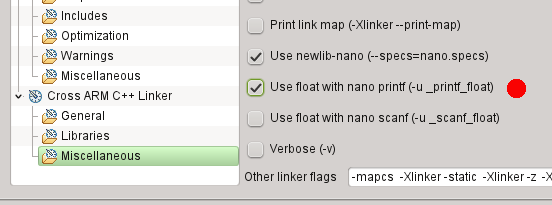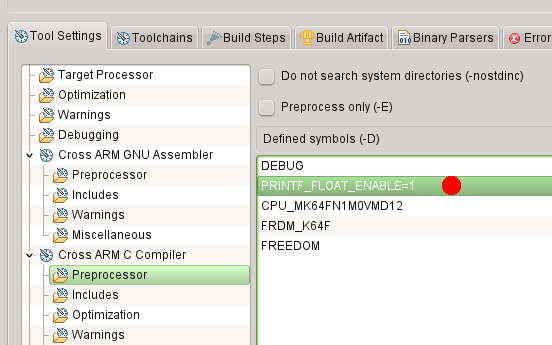## sprintf, PRINTF, DbgConsole_Printf with float and double

cancel
Showing results for
Did you mean:

## sprintf, PRINTF, DbgConsole_Printf with float and double

2,081 ViewsContributor I

I am using Kinetis Design Studio 3.2.0 with SDK 2.0 for the K64F development board.

After browsing a lot of confusing and conflicting information and some testing, I found the following concerning printf() with float and double values.

sprintf() comes from the newlib-nano, and in order to  activate floating point I need to set the following linker flag as described in many places:The very useful debug console also has a PRINTF function, that maps to DbgConsole_Printf, which is implemented separately and independently. In order to activate its floating point option, one needs to define PRINTF_FLOAT_ENABLE:The test program included below furthermore revealed a bug in the PRINTF=DbgConsole_Printf implementation:

The bit pattern 0xffffffff interpreted as a 32-bit signed integer should print as "-1" but wrongly prints as "1" instead.

Hope this helps some others to save time,

G

#include "fsl_debug_console.h"
#include "board.h"
#include "pin_mux.h"
#include "clock_config.h"
#include <math.h>
#include <stdio.h>

int main(void)
{
uint8_t u8 = 0xffU;
uint16_t u16 = 0xffffU;
uint32_t u32 = 0xffffffffU;

int8_t i8 = 100U;
int16_t i16 = 20000U;
int32_t i32 = 1000000000U;

float f = sqrtf(2.0);
double d = sqrt(2.0);
char s;

BOARD_InitPins();
BOARD_BootClockRUN();
BOARD_InitDebugConsole();

PRINTF("\r\nprintf test\r\n\r\n");
PRINTF("Using PRINTF = DbgConsole_Printf:\r\n\r\n");
PRINTF("u8 = %u = %x\r\n", (unsigned int) u8, (unsigned int) u8);
PRINTF("u16 = %u = %x\r\n", (unsigned int) u16, (unsigned int) u16);
PRINTF("u32 = %u = %x\r\n", (unsigned int) u32, (unsigned int) u32);
PRINTF("u8 as integer = %d\r\n", (int) u8);
PRINTF("u16 as integer = %d\r\n", (int) u16);
PRINTF("u32 as integer = %d\r\n", (int) u32);
PRINTF("i8 = %d\r\n", (int) i8);
PRINTF("i16 = %d\r\n", (int) i16);
PRINTF("i32 = %d\r\n", (int) i32);
PRINTF("negative i8 = %d\r\n", (int) -i8);
PRINTF("negative i16 = %d\r\n", (int) -i16);
PRINTF("negative i32 = %d\r\n", (int) -i32);
PRINTF("float = %.16f\r\n", f);
PRINTF("double = %.16f\r\n", d);

PRINTF("\r\nUsing sprintf:\r\n\r\n");
sprintf(s, "u8 = %u = %x\r\n", (unsigned int) u8, (unsigned int) u8);
PRINTF("%s", s);
sprintf(s, "u16 = %u = %x\r\n", (unsigned int) u16, (unsigned int) u16);
PRINTF("%s", s);
sprintf(s, "u32 = %u = %x\r\n", (unsigned int) u32, (unsigned int) u32);
PRINTF("%s", s);
sprintf(s, "u8 as integer = %d\r\n", (int) u8);
PRINTF("%s", s);
sprintf(s, "u16 as integer = %d\r\n", (int) u16);
PRINTF("%s", s);
sprintf(s, "u32 as integer = %d\r\n", (int) u32);
PRINTF("%s", s);
sprintf(s, "i8 = %d\r\n", (int) i8);
PRINTF("%s", s);
sprintf(s, "i16 = %d\r\n", (int) i16);
PRINTF("%s", s);
sprintf(s, "i32 = %d\r\n", (int) i32);
PRINTF("%s", s);
sprintf(s, "negative i8 = %d\r\n", (int) -i8);
PRINTF("%s", s);
sprintf(s, "negative i16 = %d\r\n", (int) -i16);
PRINTF("%s", s);
sprintf(s, "negative i32 = %d\r\n", (int) -i32);
PRINTF("%s", s);
sprintf(s, "float = %.16f\r\n", f);
PRINTF("%s", s);
sprintf(s, "double = %.16f\r\n", d);
PRINTF("%s", s);

while (1)
;
return 0;
}

Tags (5)
0 Replies